Скачать презентацию Preliminaries Mathematical Preliminaries Strings and Languages 1

b55ae347ad6b52fa923398176bd8d4ee.ppt

• Количество слайдов: 65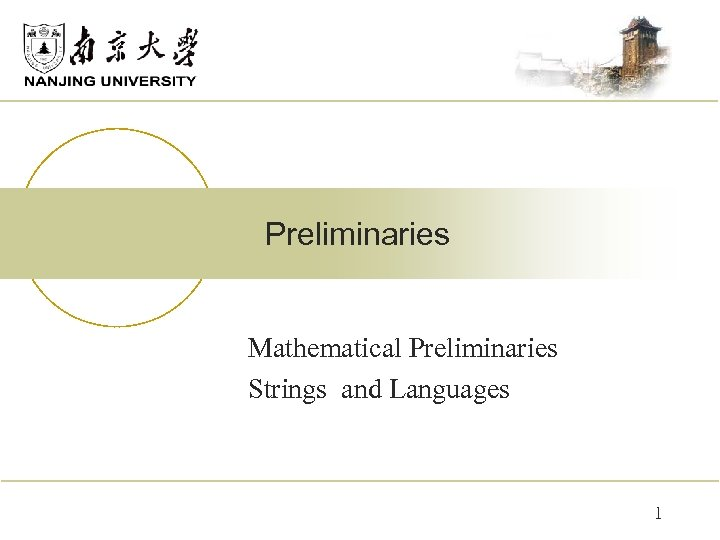Preliminaries Mathematical Preliminaries Strings and Languages 1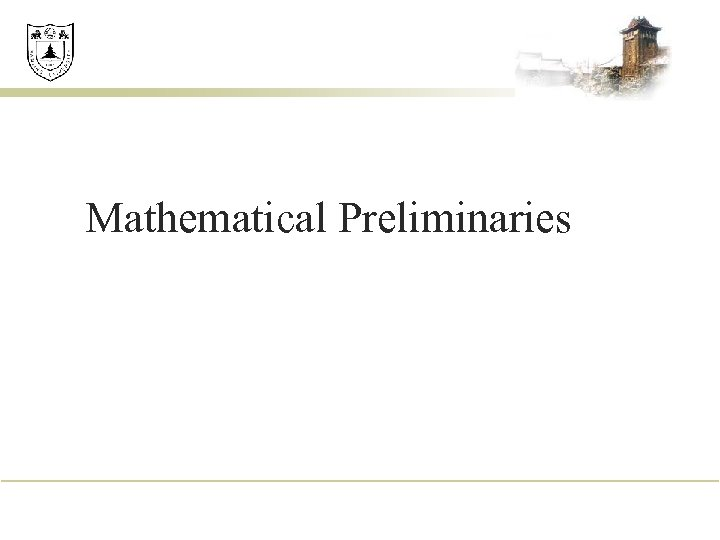Mathematical Preliminaries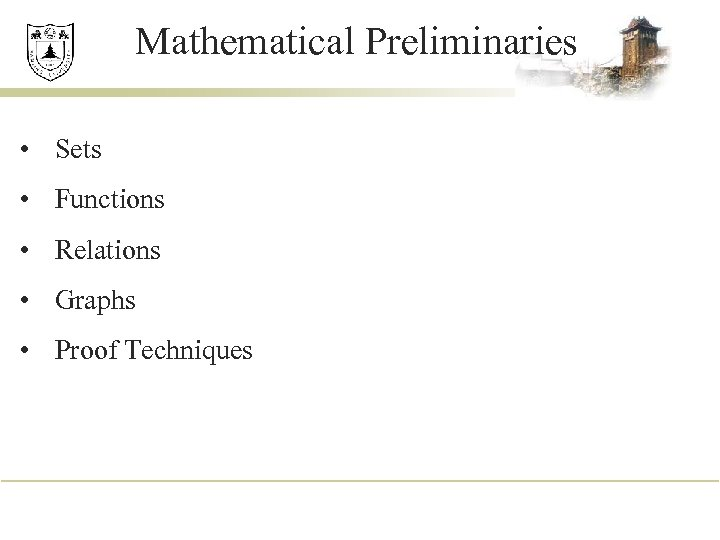Mathematical Preliminaries • Sets • Functions • Relations • Graphs • Proof Techniques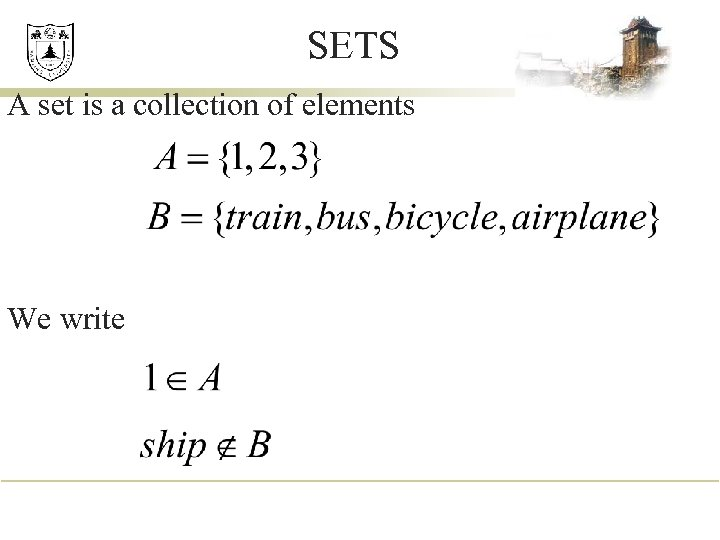SETS A set is a collection of elements We write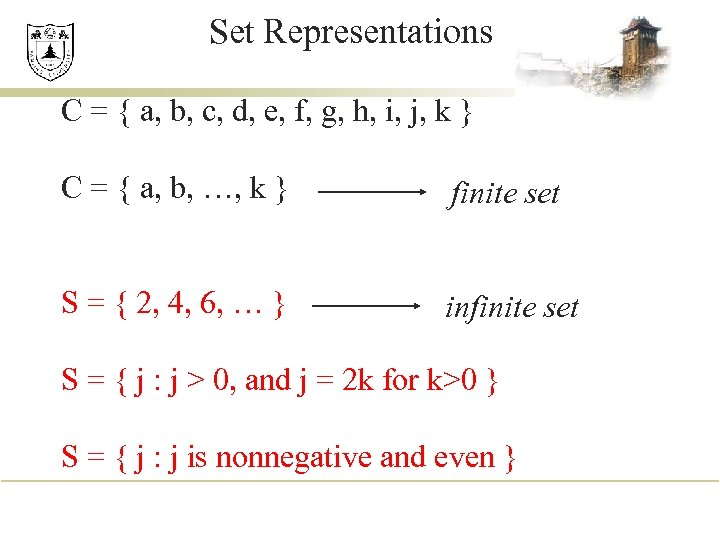Set Representations C = { a, b, c, d, e, f, g, h, i, j, k } C = { a, b, …, k } finite set S = { 2, 4, 6, … } infinite set S = { j : j > 0, and j = 2 k for k>0 } S = { j : j is nonnegative and even }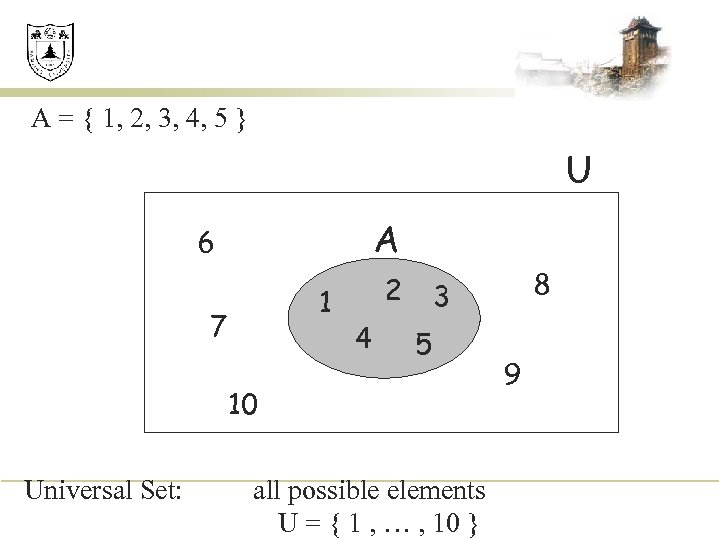A = { 1, 2, 3, 4, 5 } U A 6 1 7 2 4 5 10 Universal Set: 8 3 all possible elements U = { 1 , … , 10 } 9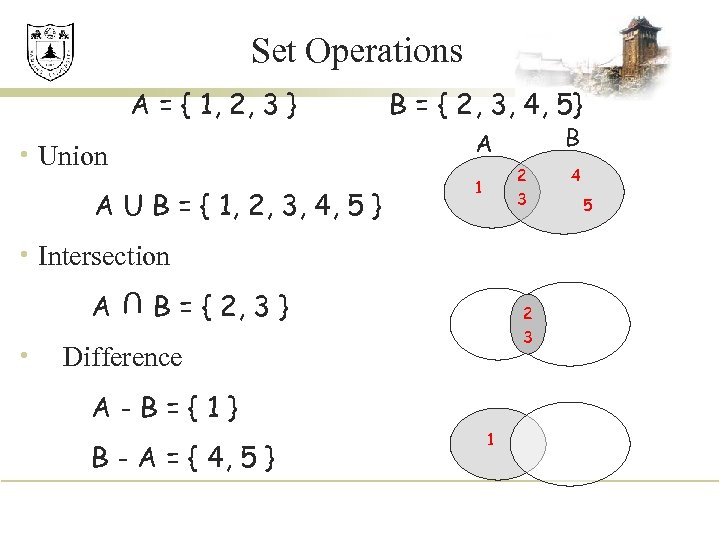Set Operations A = { 1, 2, 3 } B = { 2, 3, 4, 5} B A • Union A U B = { 1, 2, 3, 4, 5 } 2 3 1 • Intersection • U A B = { 2, 3 } 2 3 Difference A-B={1} B - A = { 4, 5 } 1 4 5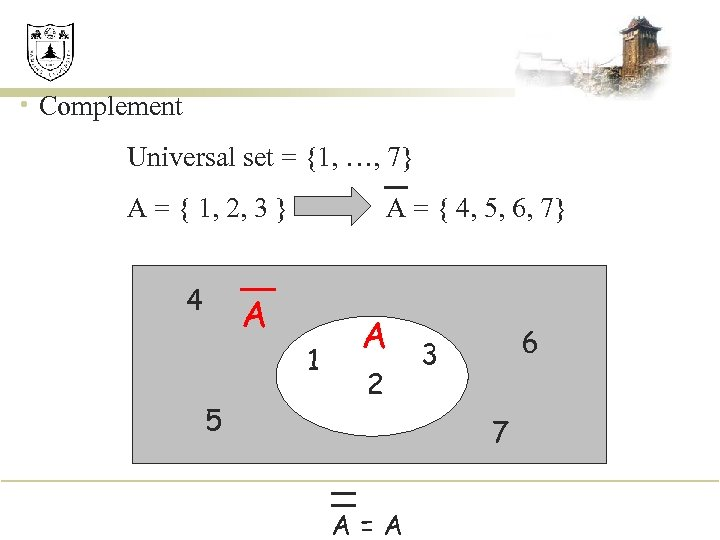• Complement Universal set = {1, …, 7} A = { 1, 2, 3 } 4 A = { 4, 5, 6, 7} A 1 5 A 2 6 3 7 A=A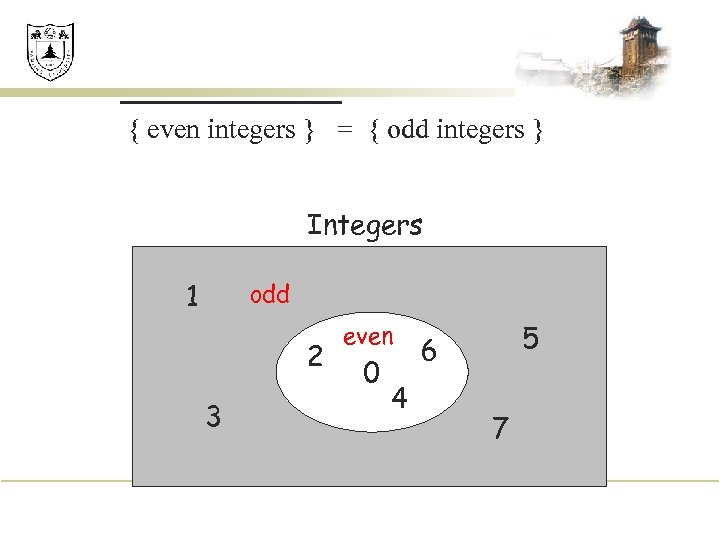{ even integers } = { odd integers } Integers 1 odd 2 3 even 0 4 5 6 7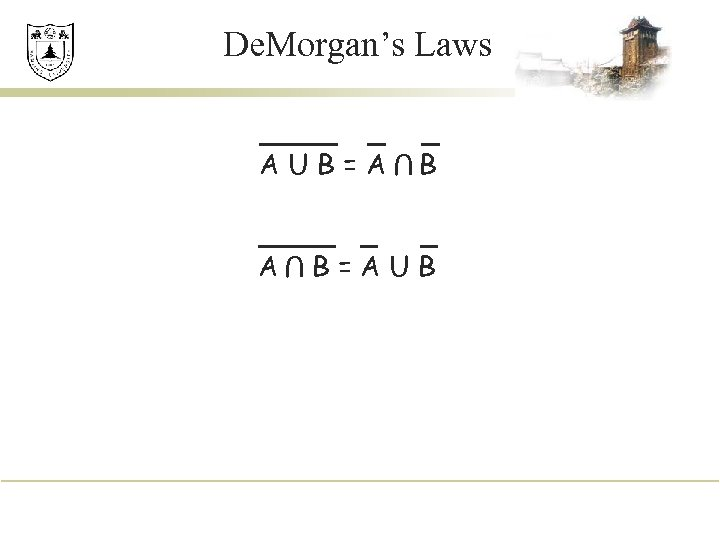De. Morgan’s Laws U AUB=A B B=AUB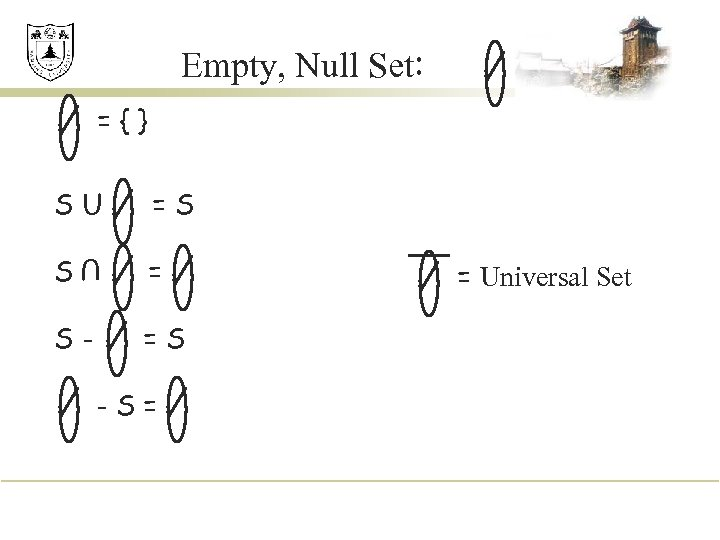Empty, Null Set: ={} SU =S S = U S- =S -S= = Universal Set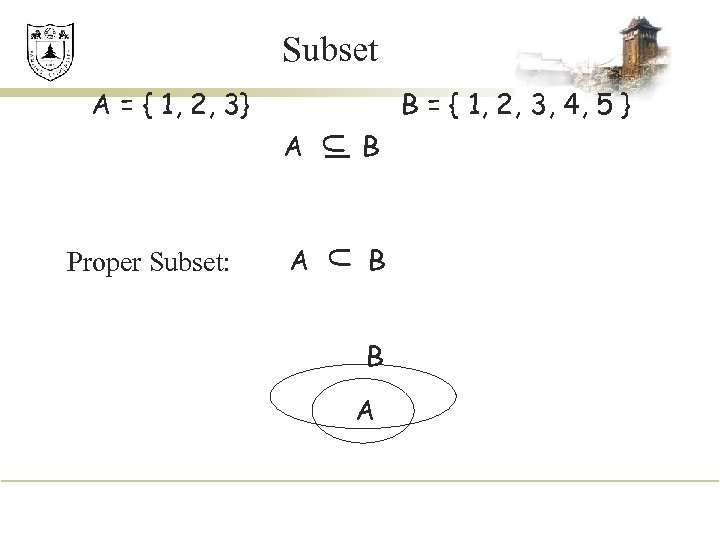Subset A = { 1, 2, 3} B = { 1, 2, 3, 4, 5 } A B U Proper Subset: U A B B A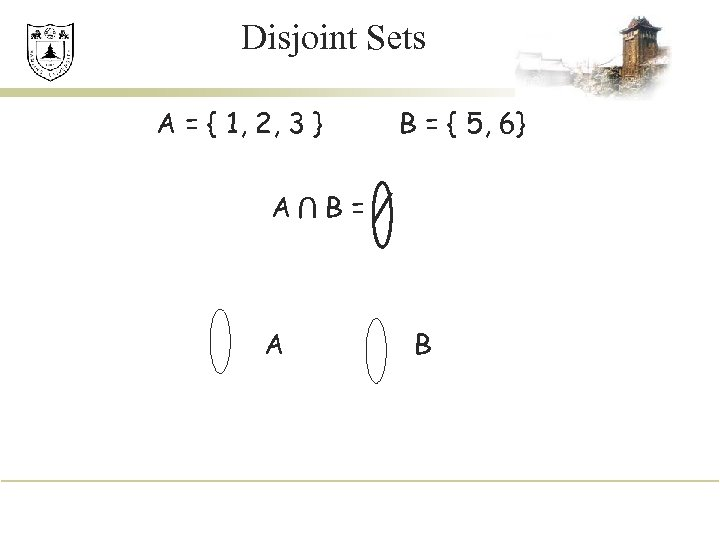Disjoint Sets A = { 1, 2, 3 } A U A B = { 5, 6} B= B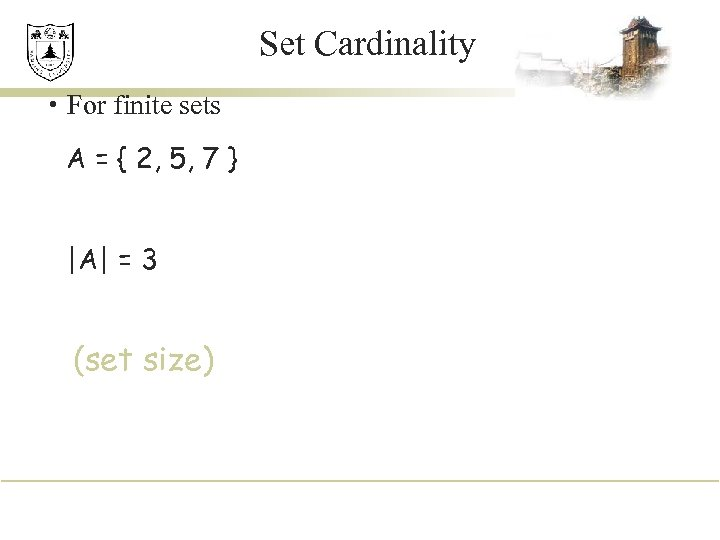Set Cardinality • For finite sets A = { 2, 5, 7 } |A| = 3 (set size)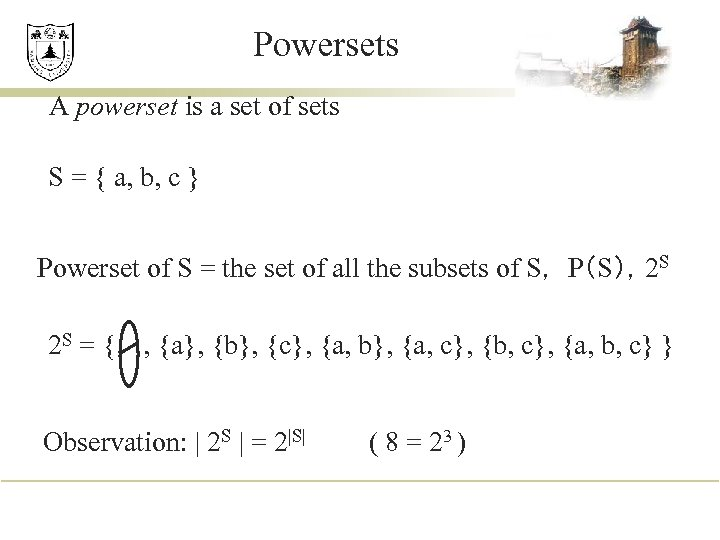Powersets A powerset is a set of sets S = { a, b, c } Powerset of S = the set of all the subsets of S， P（S），2 S 2 S = { , {a}, {b}, {c}, {a, b}, {a, c}, {b, c}, {a, b, c} } Observation: | 2 S | = 2|S| ( 8 = 23 )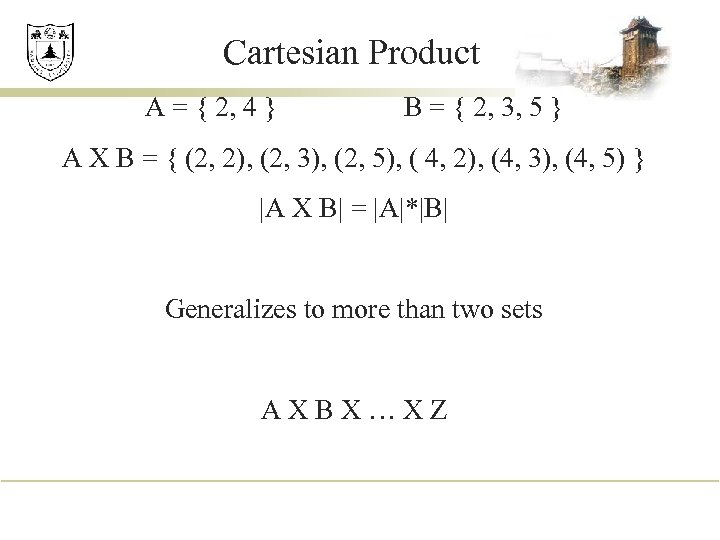Cartesian Product A = { 2, 4 } B = { 2, 3, 5 } A X B = { (2, 2), (2, 3), (2, 5), ( 4, 2), (4, 3), (4, 5) } |A X B| = |A|*|B| Generalizes to more than two sets AXBX…XZ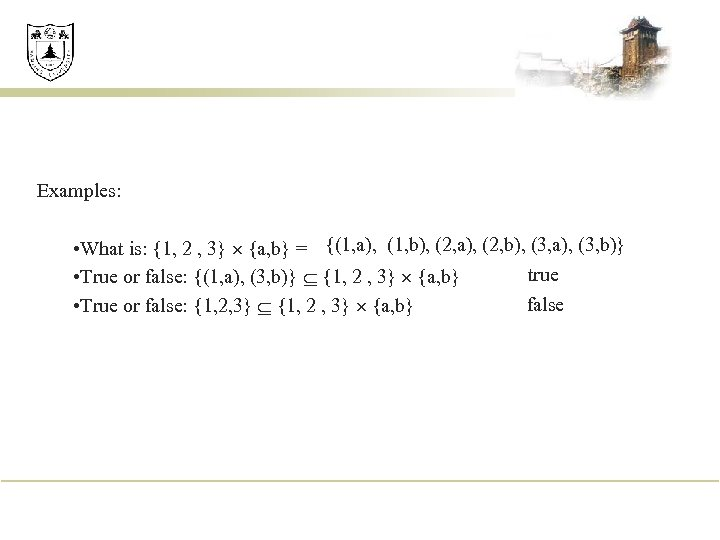Examples: • What is: {1, 2 , 3} {a, b} = {(1, a), (1, b), (2, a), (2, b), (3, a), (3, b)} true • True or false: {(1, a), (3, b)} {1, 2 , 3} {a, b} false • True or false: {1, 2, 3} {1, 2 , 3} {a, b}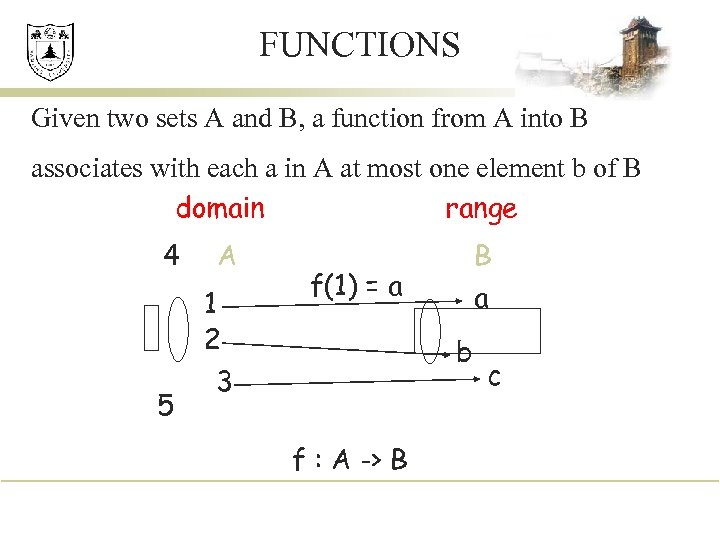FUNCTIONS Given two sets A and B, a function from A into B associates with each a in A at most one element b of B range domain 4 5 A 1 2 3 B a f(1) = a b f : A -> B c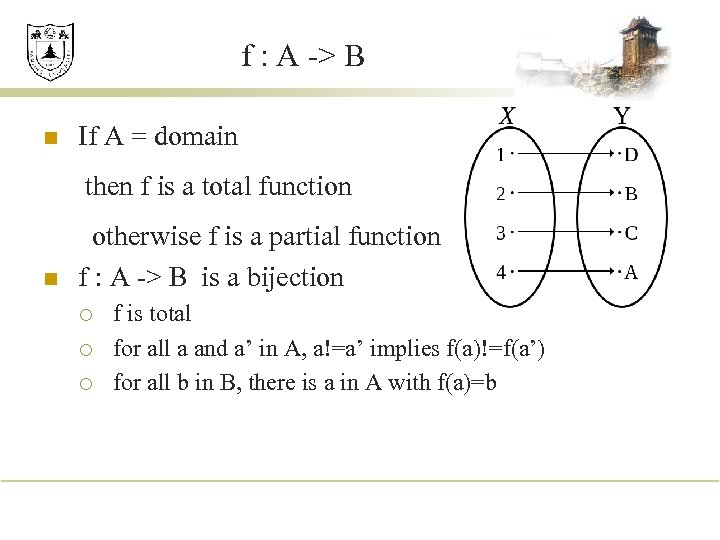f : A -> B n If A = domain then f is a total function n otherwise f is a partial function f : A -> B is a bijection ¡ ¡ ¡ f is total for all a and a’ in A, a!=a’ implies f(a)!=f(a’) for all b in B, there is a in A with f(a)=b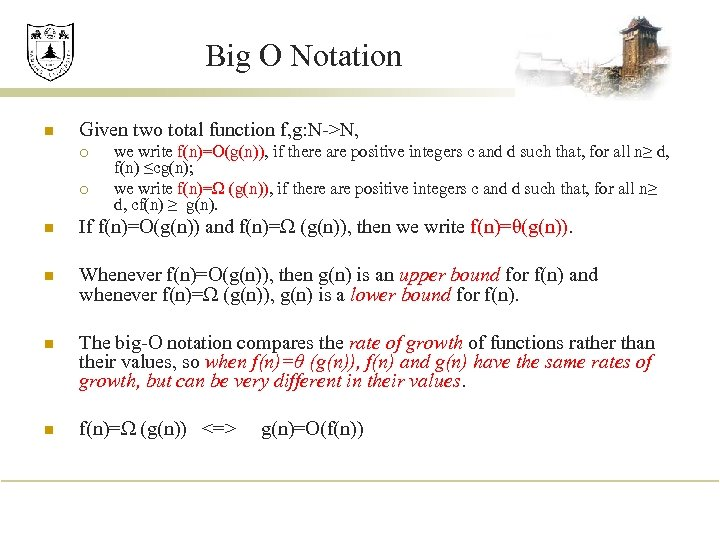Big O Notation n Given two total function f, g: N->N, ¡ ¡ we write f(n)=O(g(n)), if there are positive integers c and d such that, for all n≥ d, f(n) ≤cg(n); we write f(n)=Ω (g(n)), if there are positive integers c and d such that, for all n≥ d, cf(n) ≥ g(n). n If f(n)=O(g(n)) and f(n)=Ω (g(n)), then we write f(n)=θ(g(n)). n Whenever f(n)=O(g(n)), then g(n) is an upper bound for f(n) and whenever f(n)=Ω (g(n)), g(n) is a lower bound for f(n). n The big-O notation compares the rate of growth of functions rather than their values, so when f(n)=θ (g(n)), f(n) and g(n) have the same rates of growth, but can be very different in their values. n f(n)=Ω (g(n)) <=> g(n)=O(f(n))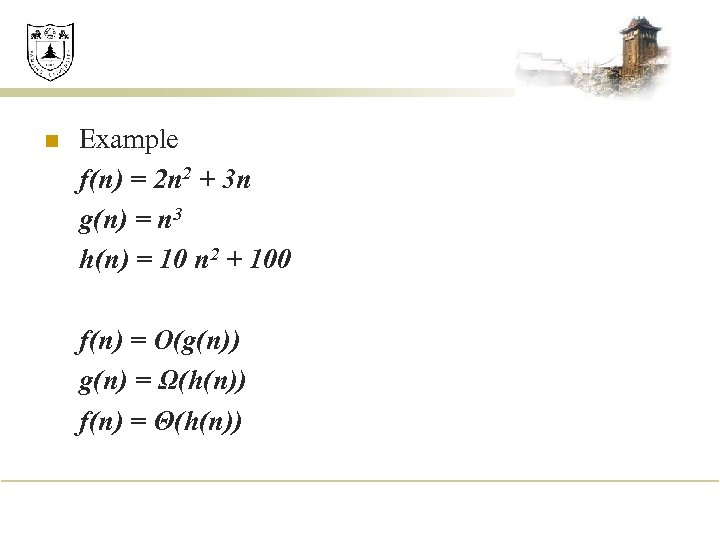n Example f(n) = 2 n 2 + 3 n g(n) = n 3 h(n) = 10 n 2 + 100 f(n) = O(g(n)) g(n) = Ω(h(n)) f(n) = Θ(h(n))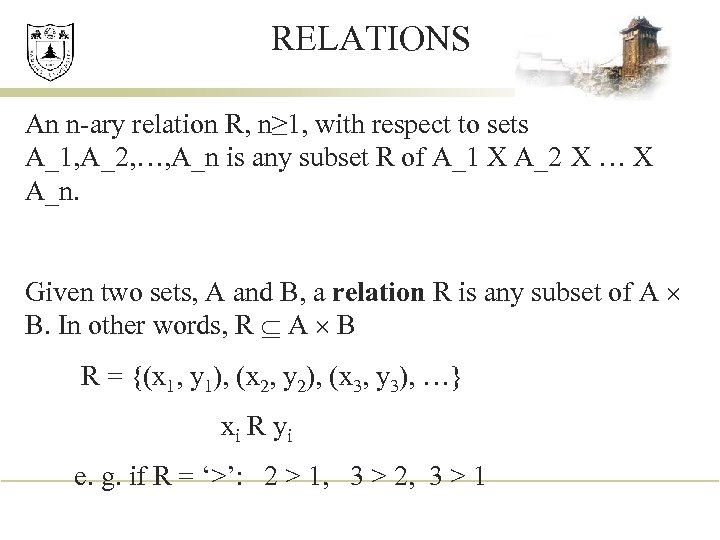RELATIONS An n-ary relation R, n≥ 1, with respect to sets A_1, A_2, …, A_n is any subset R of A_1 X A_2 X … X A_n. Given two sets, A and B, a relation R is any subset of A B. In other words, R A B R = {(x 1, y 1), (x 2, y 2), (x 3, y 3), …} xi R yi e. g. if R = ‘>’: 2 > 1, 3 > 2, 3 > 1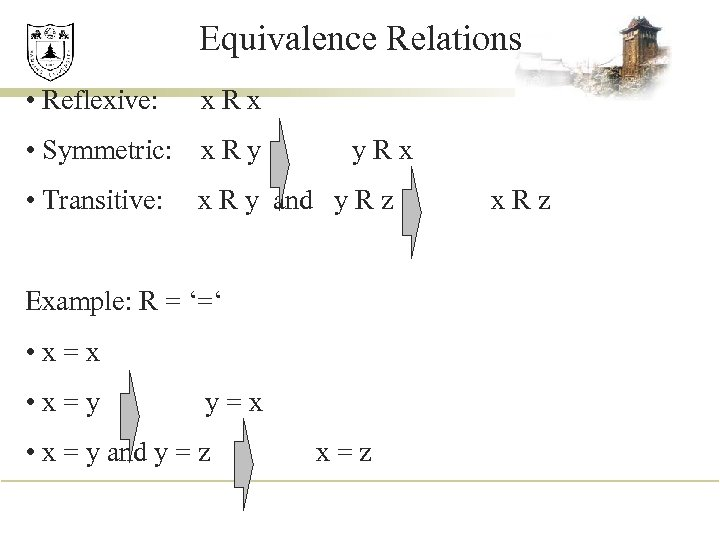Equivalence Relations • Reflexive: x. Rx • Symmetric: x R y • Transitive: y. Rx x R y and y R z Example: R = ‘=‘ • x=x • x=y y=x • x = y and y = z x=z x. Rz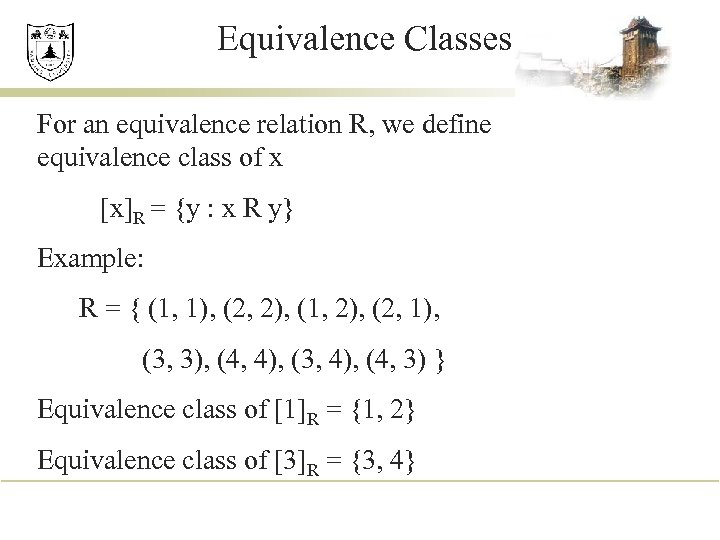Equivalence Classes For an equivalence relation R, we define equivalence class of x [x]R = {y : x R y} Example: R = { (1, 1), (2, 2), (1, 2), (2, 1), (3, 3), (4, 4), (3, 4), (4, 3) } Equivalence class of R = {1, 2} Equivalence class of R = {3, 4}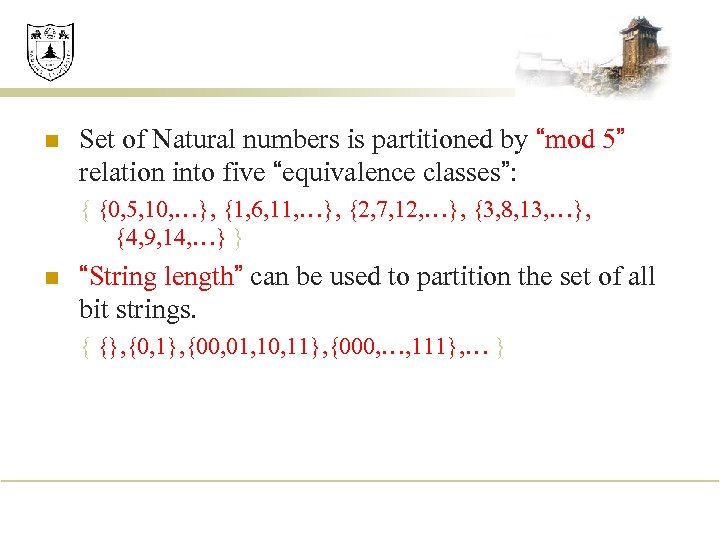n Set of Natural numbers is partitioned by “mod 5” relation into five “equivalence classes”: { {0, 5, 10, …}, {1, 6, 11, …}, {2, 7, 12, …}, {3, 8, 13, …}, {4, 9, 14, …} } n “String length” can be used to partition the set of all bit strings. { {}, {0, 1}, {00, 01, 10, 11}, {000, …, 111}, … }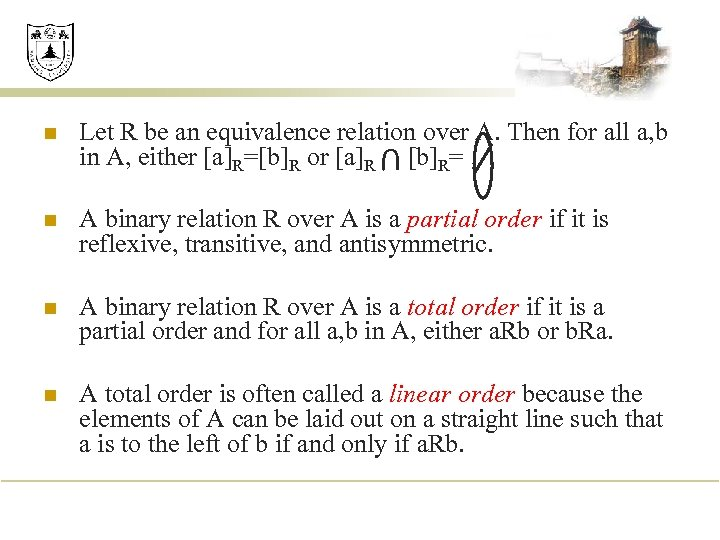n Let R be an equivalence relation over A. Then for all a, b in A, either [a]R=[b]R or [a]R [b]R= n A binary relation R over A is a partial order if it is reflexive, transitive, and antisymmetric. n A binary relation R over A is a total order if it is a partial order and for all a, b in A, either a. Rb or b. Ra. n A total order is often called a linear order because the elements of A can be laid out on a straight line such that a is to the left of b if and only if a. Rb. U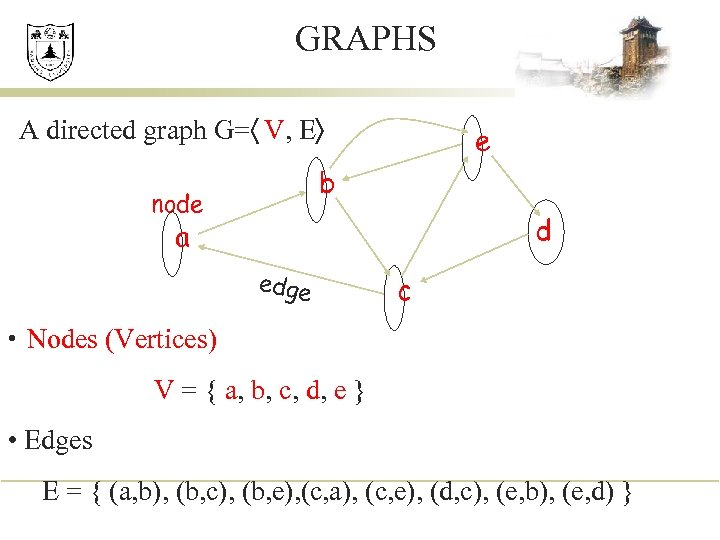GRAPHS A directed graph G=〈 V, E〉 e b node d a edge c • Nodes (Vertices) V = { a, b, c, d, e } • Edges E = { (a, b), (b, c), (b, e), (c, a), (c, e), (d, c), (e, b), (e, d) }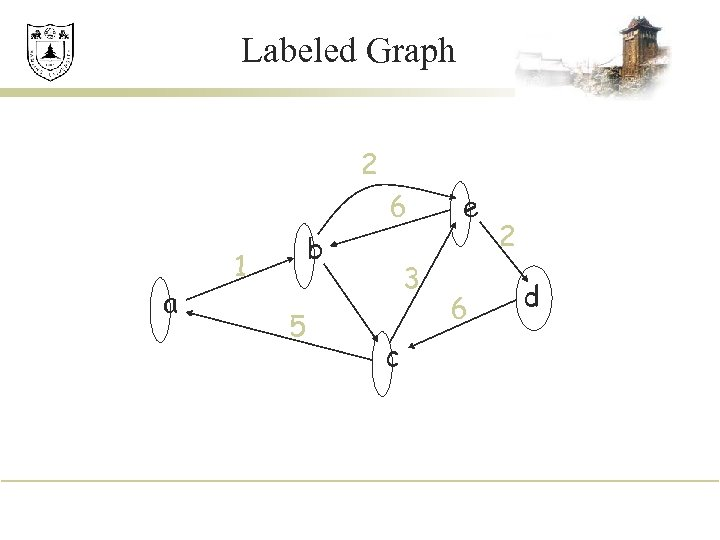Labeled Graph 2 6 a b 1 5 3 c e 6 2 d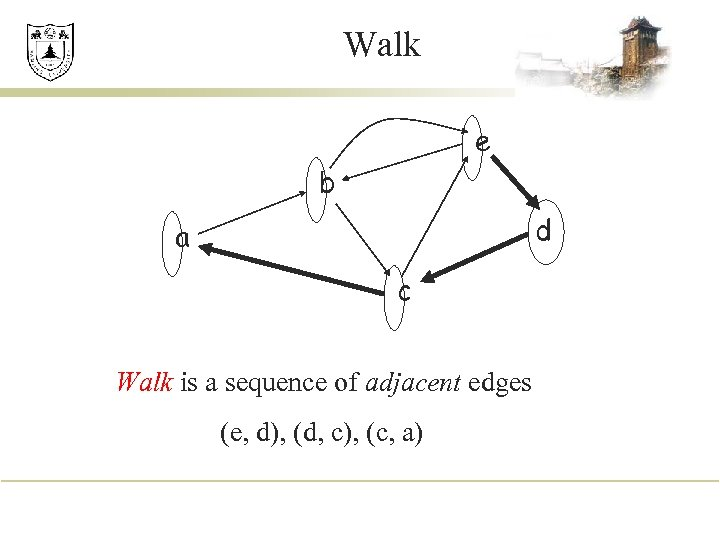Walk e b d a c Walk is a sequence of adjacent edges (e, d), (d, c), (c, a)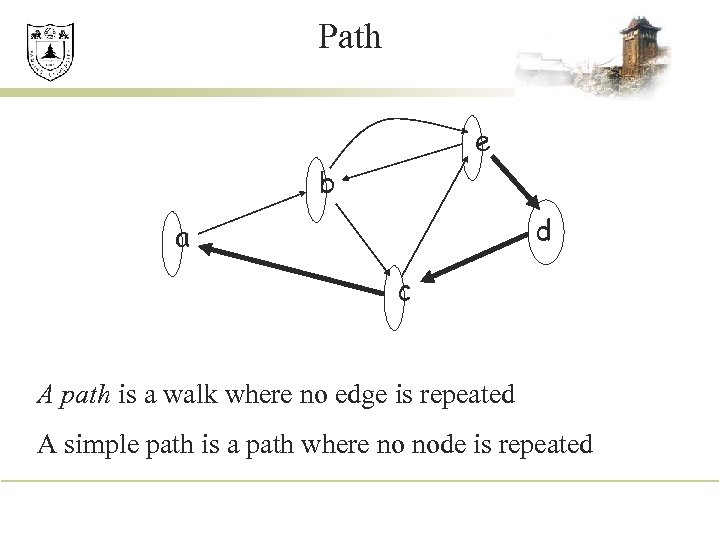Path e b d a c A path is a walk where no edge is repeated A simple path is a path where no node is repeated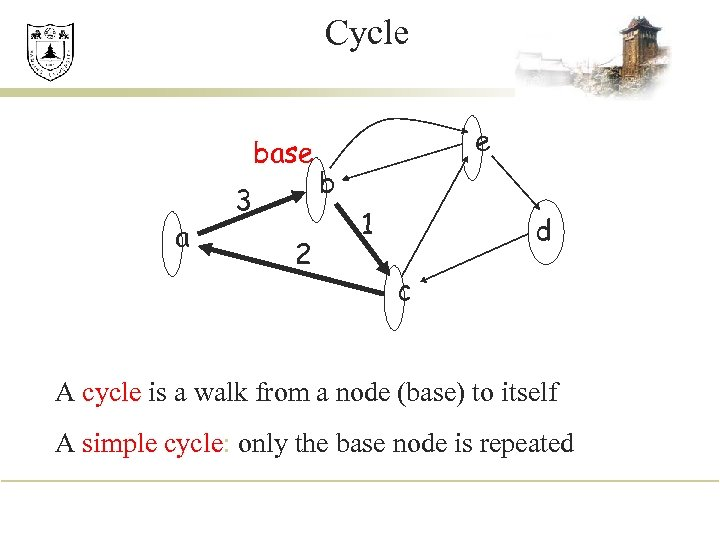Cycle base a 3 2 e b 1 d c A cycle is a walk from a node (base) to itself A simple cycle: only the base node is repeated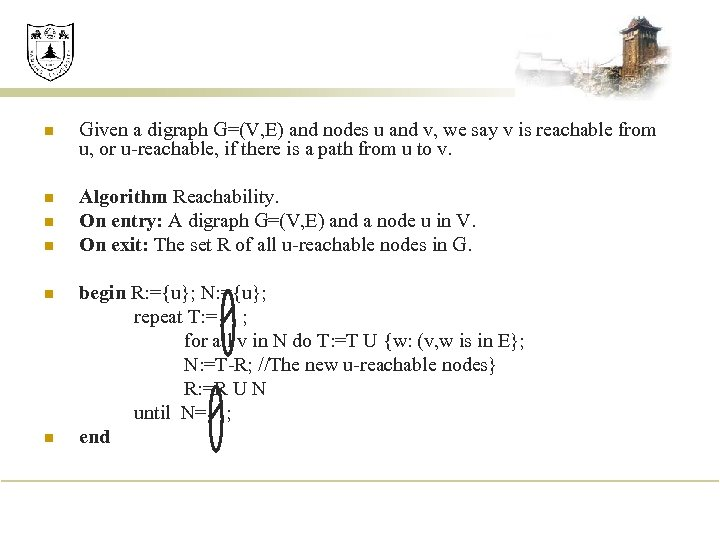n Given a digraph G=(V, E) and nodes u and v, we say v is reachable from u, or u-reachable, if there is a path from u to v. n Algorithm Reachability. On entry: A digraph G=(V, E) and a node u in V. On exit: The set R of all u-reachable nodes in G. n n begin R: ={u}; N: ={u}; repeat T: = ; for all v in N do T: =T U {w: (v, w is in E}; N: =T-R; //The new u-reachable nodes} R: =R U N until N= ; end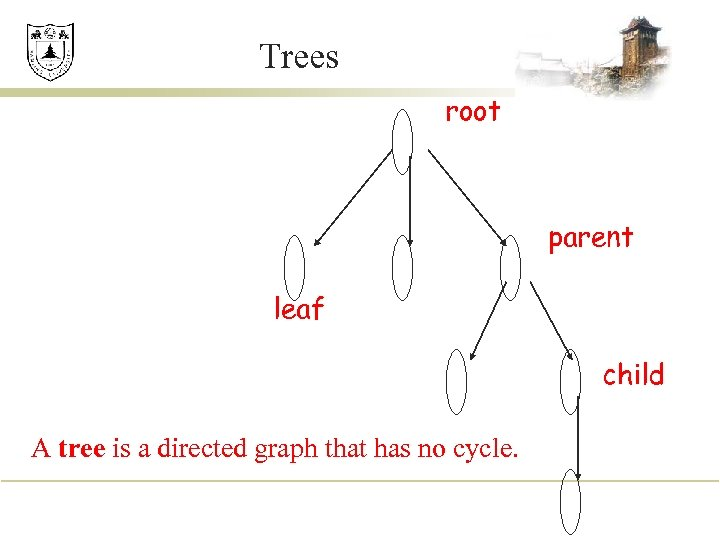Trees root parent leaf child A tree is a directed graph that has no cycle.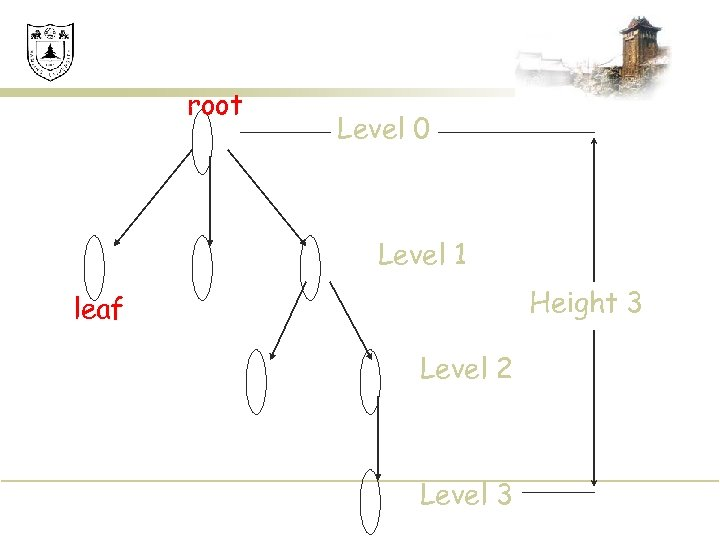root Level 0 Level 1 Height 3 leaf Level 2 Level 3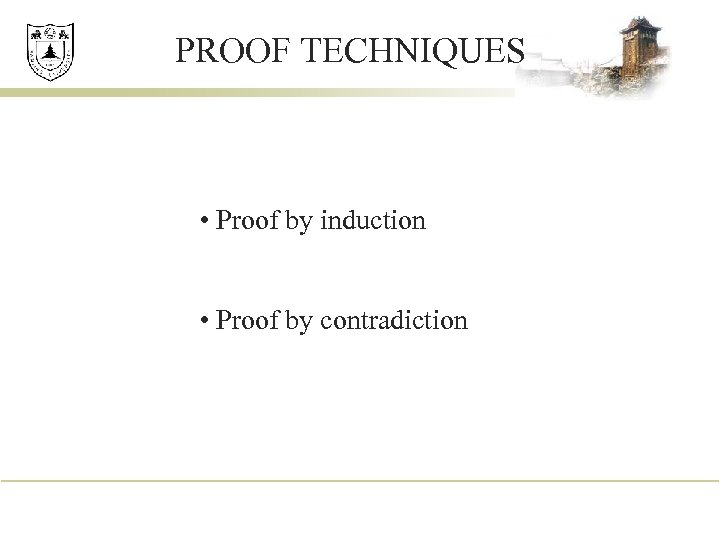PROOF TECHNIQUES • Proof by induction • Proof by contradiction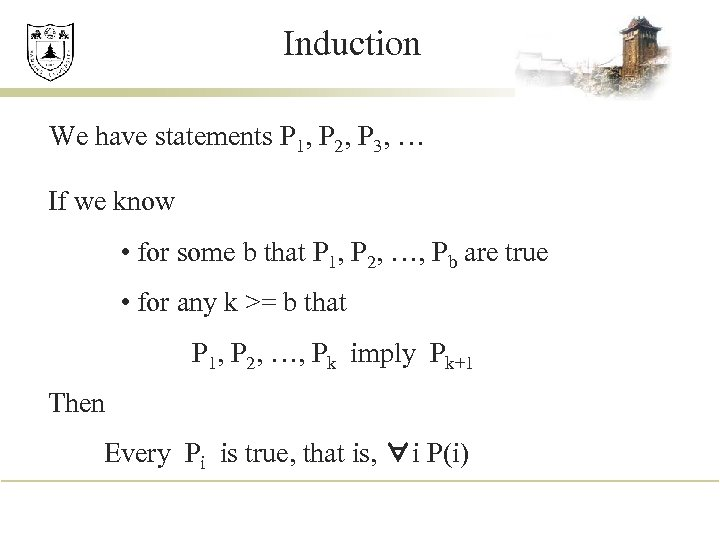Induction We have statements P 1, P 2, P 3, … If we know • for some b that P 1, P 2, …, Pb are true • for any k >= b that P 1, P 2, …, Pk imply Pk+1 Then Every Pi is true, that is, ∀i P(i)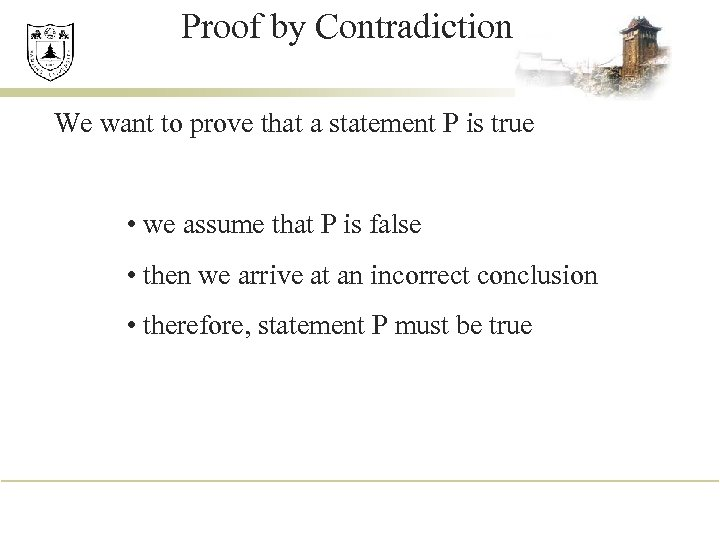Proof by Contradiction We want to prove that a statement P is true • we assume that P is false • then we arrive at an incorrect conclusion • therefore, statement P must be true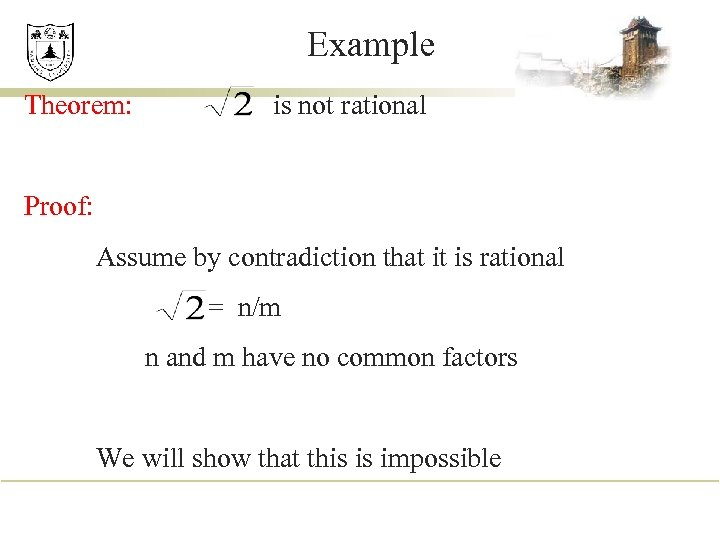Example Theorem: is not rational Proof: Assume by contradiction that it is rational = n/m n and m have no common factors We will show that this is impossible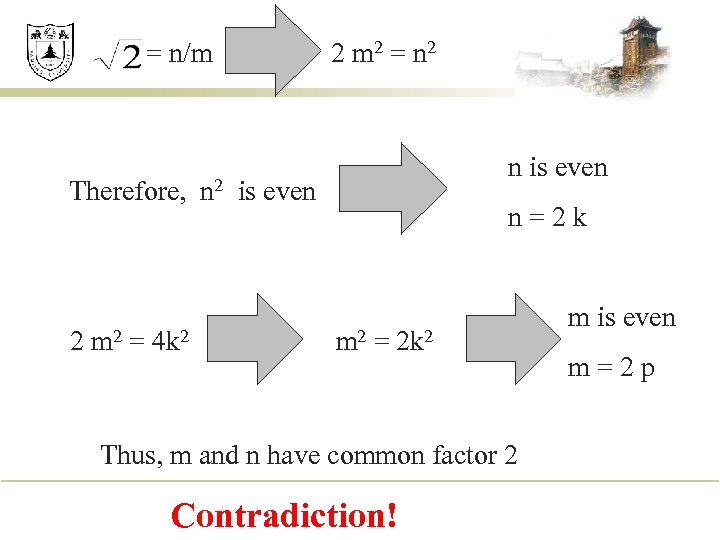= n/m Therefore, 2 m 2 = 4 k 2 n 2 2 m 2 = n 2 n is even n=2 k m 2 = 2 k 2 Thus, m and n have common factor 2 Contradiction! m is even m=2 p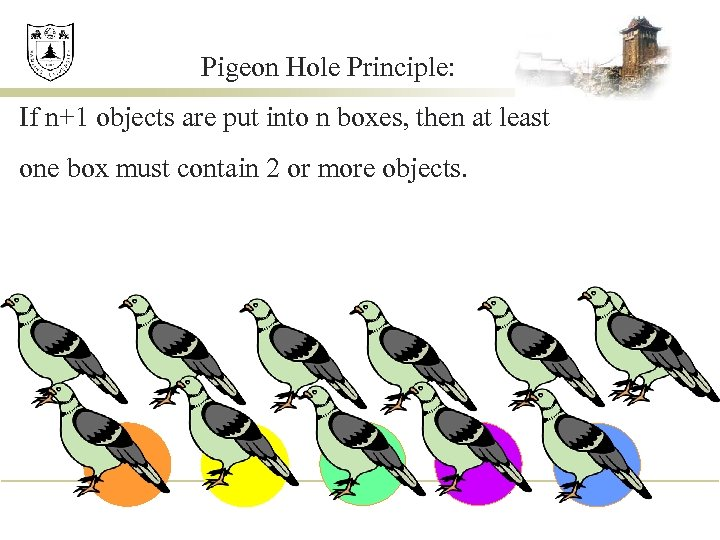Pigeon Hole Principle: If n+1 objects are put into n boxes, then at least one box must contain 2 or more objects.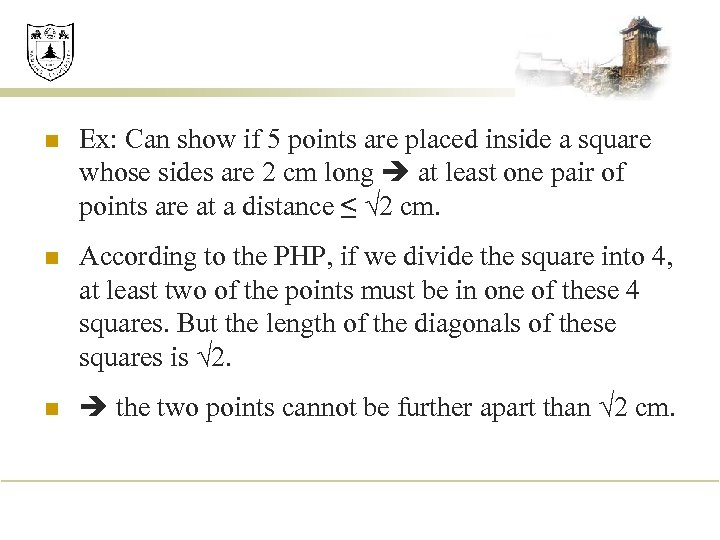n Ex: Can show if 5 points are placed inside a square whose sides are 2 cm long at least one pair of points are at a distance ≤ 2 cm. n According to the PHP, if we divide the square into 4, at least two of the points must be in one of these 4 squares. But the length of the diagonals of these squares is 2. n the two points cannot be further apart than 2 cm.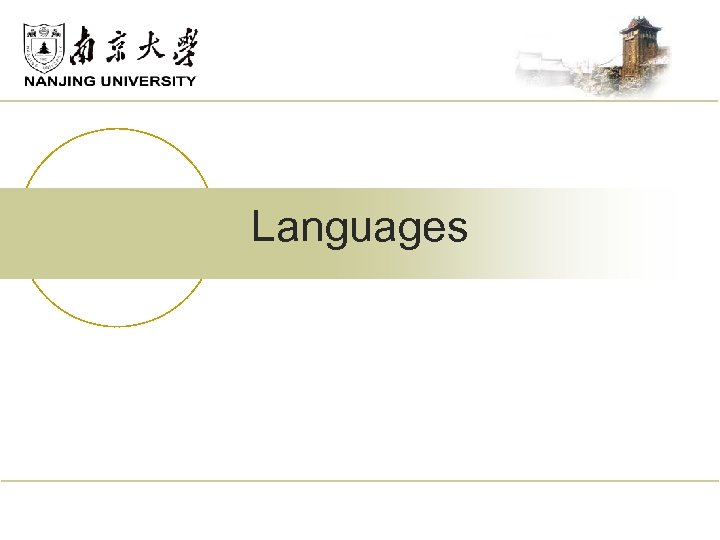Languages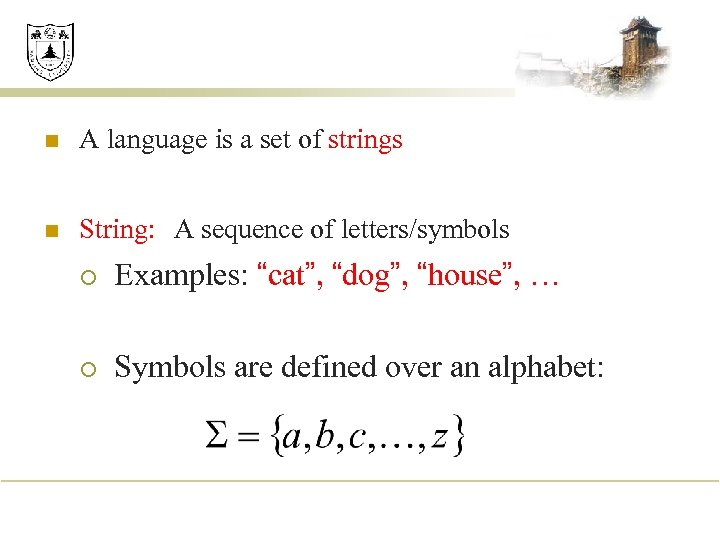n A language is a set of strings n String: A sequence of letters/symbols ¡ Examples: “cat”, “dog”, “house”, … ¡ Symbols are defined over an alphabet: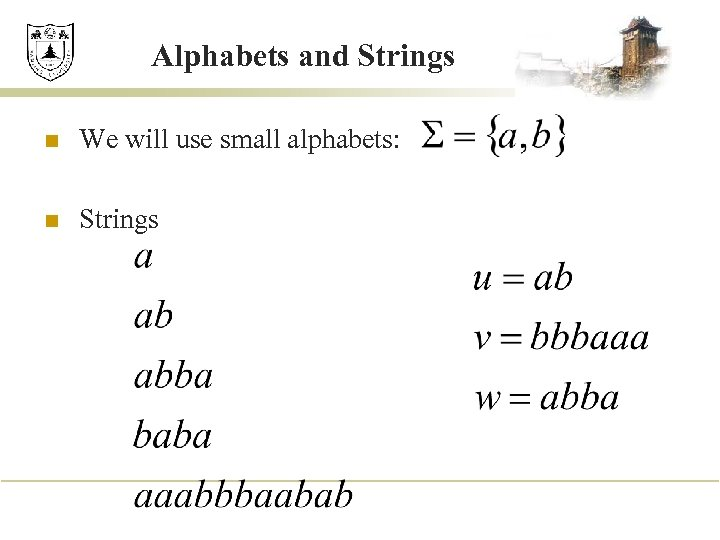Alphabets and Strings n We will use small alphabets: n Strings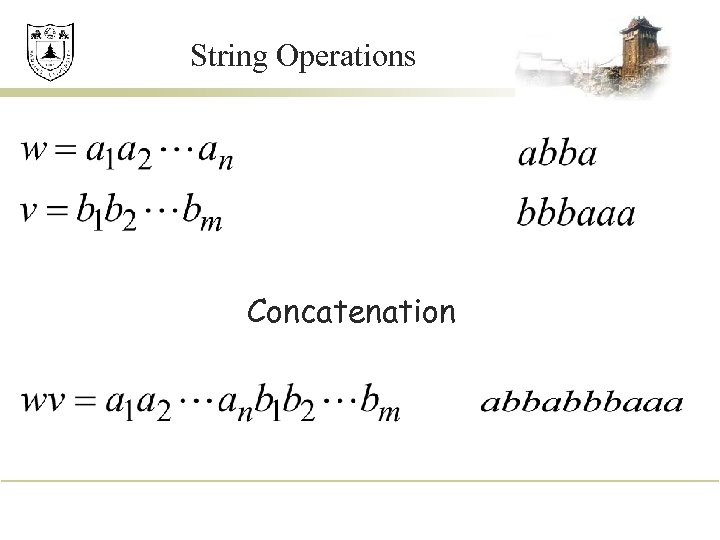String Operations Concatenation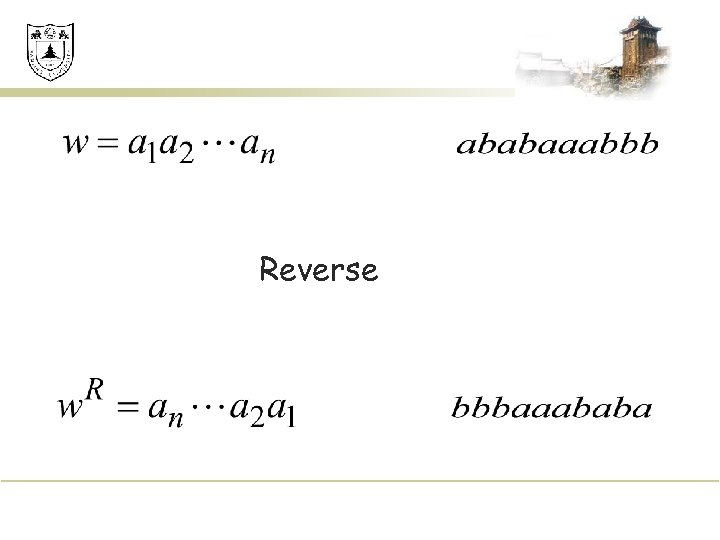Reverse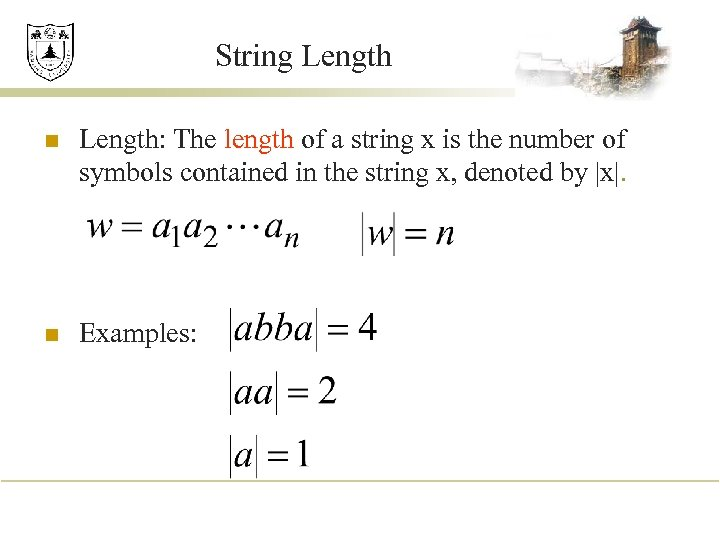String Length n Length: The length of a string x is the number of symbols contained in the string x, denoted by |x|. n Examples: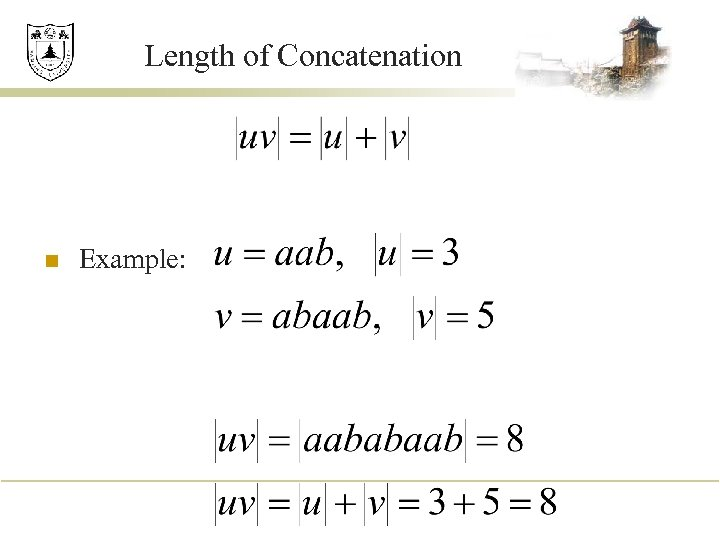Length of Concatenation n Example: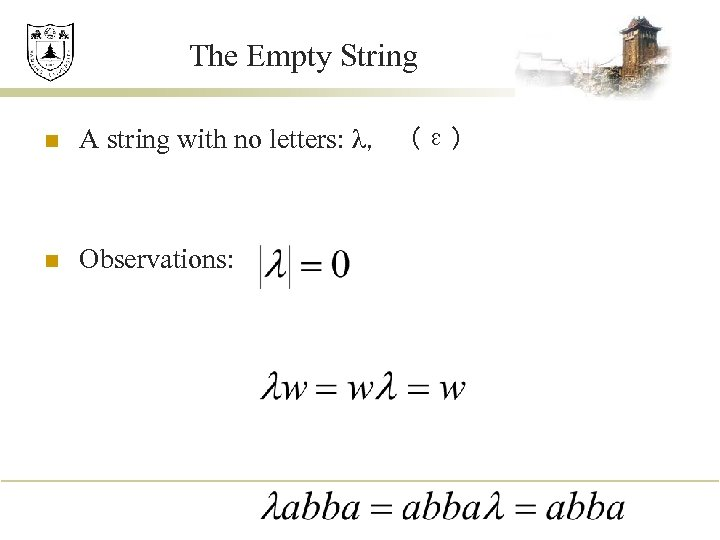The Empty String n A string with no letters: λ，（ε） n Observations: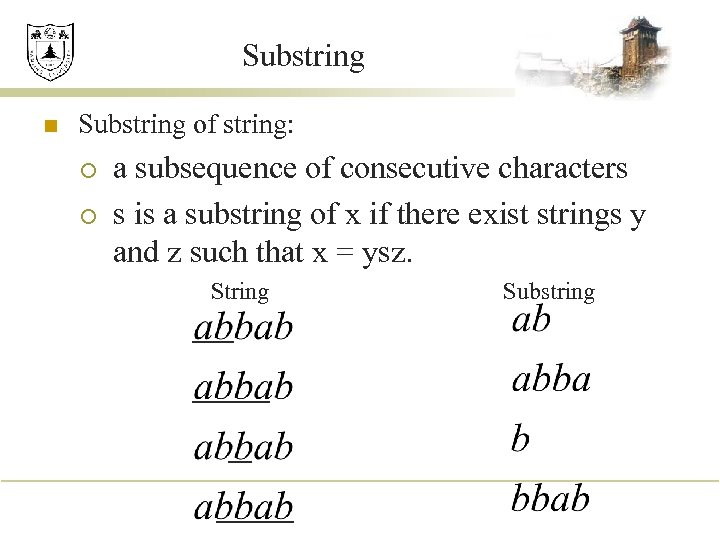Substring n Substring of string: ¡ ¡ a subsequence of consecutive characters s is a substring of x if there exist strings y and z such that x = ysz. String Substring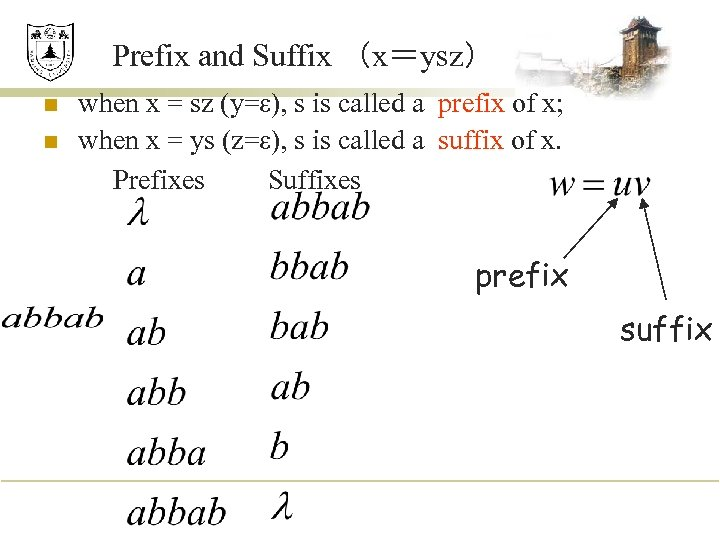Prefix and Suffix （x＝ysz） n n when x = sz (y=ε), s is called a prefix of x; when x = ys (z=ε), s is called a suffix of x. Prefixes Suffixes prefix suffix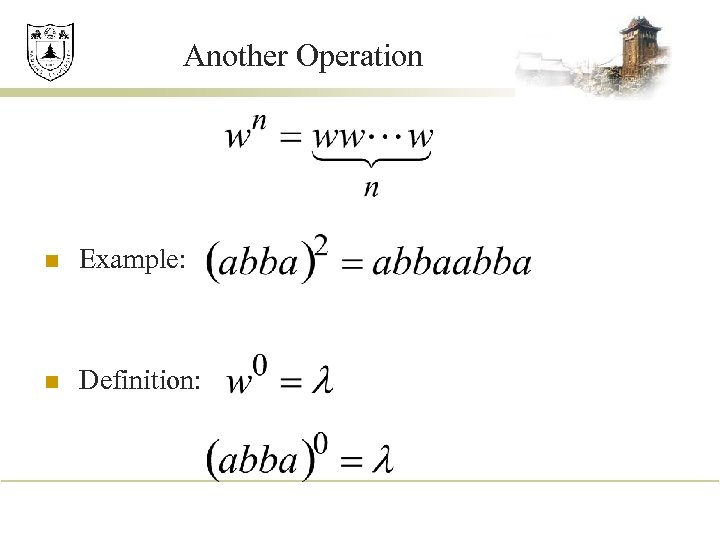Another Operation n Example: n Definition: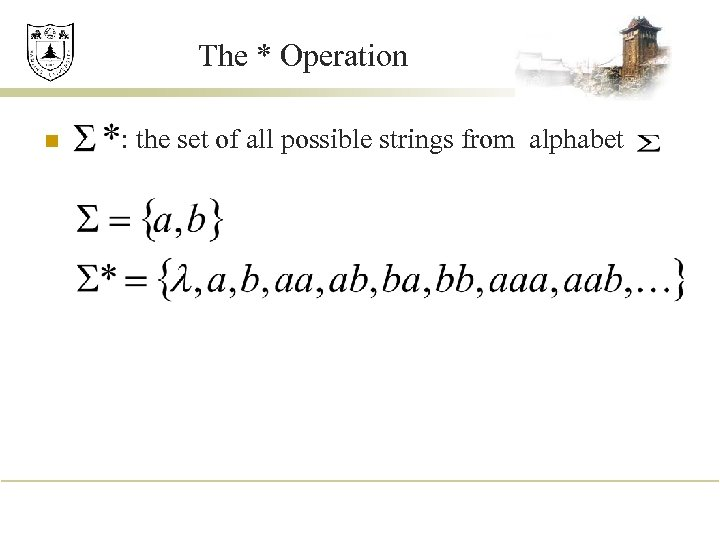The * Operation n : the set of all possible strings from alphabet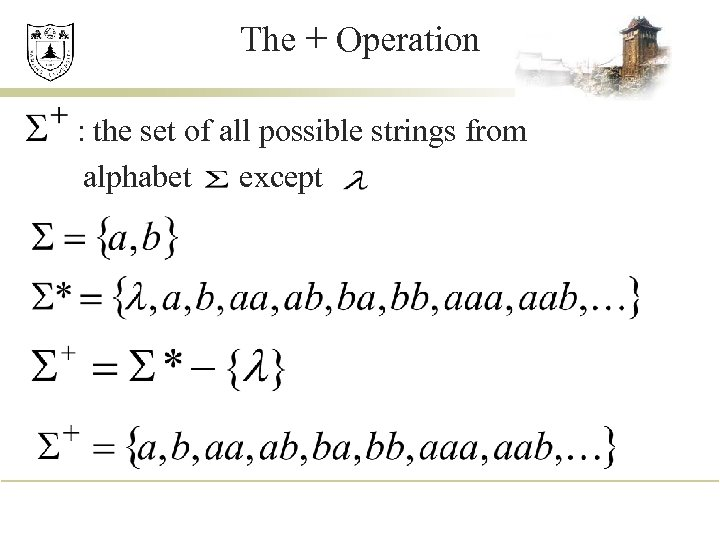The + Operation : the set of all possible strings from alphabet except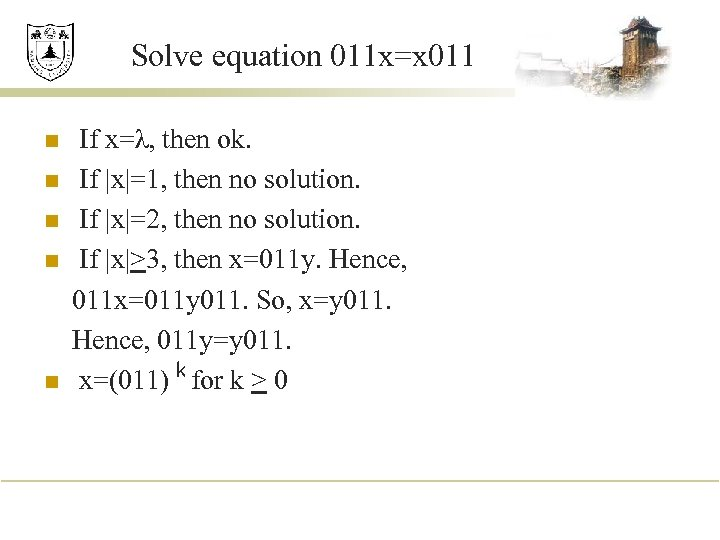Solve equation 011 x=x 011 n n n If x=λ, then ok. If |x|=1, then no solution. If |x|=2, then no solution. If |x|>3, then x=011 y. Hence, 011 x=011 y 011. So, x=y 011. Hence, 011 y=y 011. x=(011) k for k > 0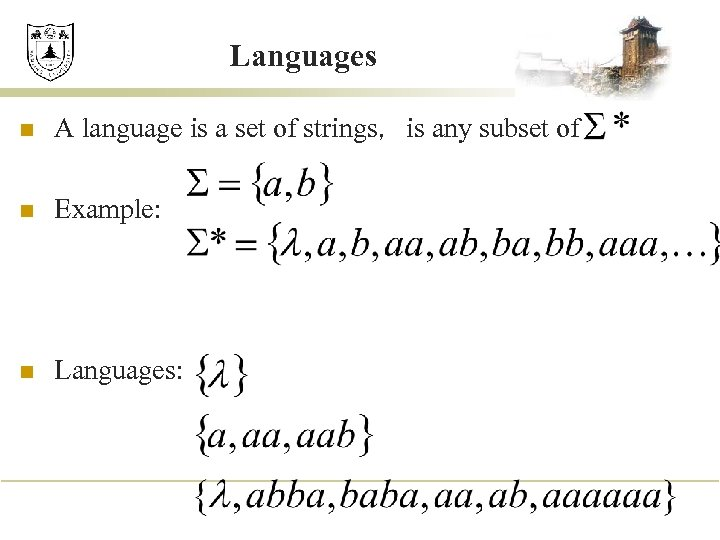Languages n A language is a set of strings，is any subset of n Example: n Languages: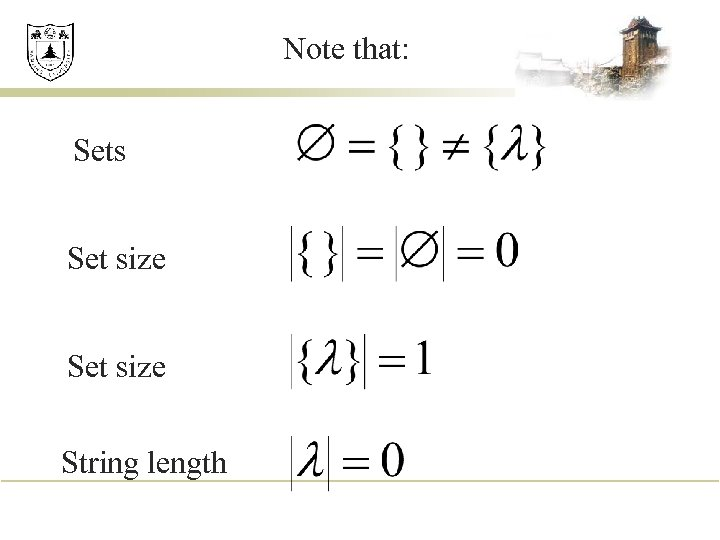Note that: Sets Set size String length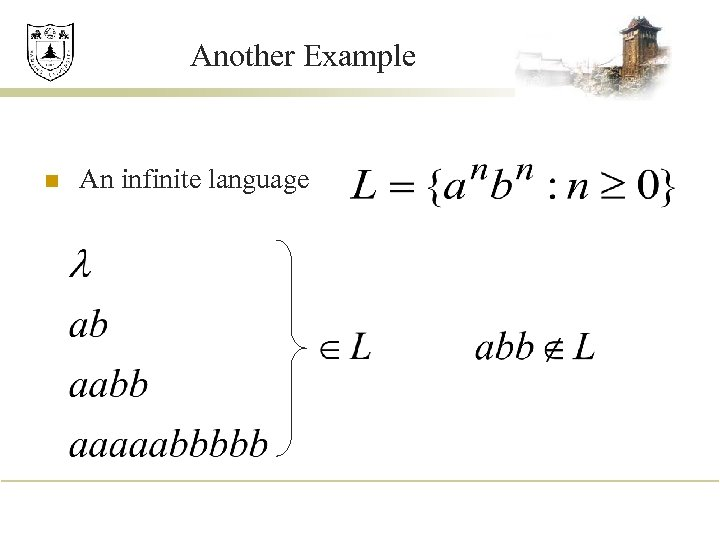Another Example n An infinite language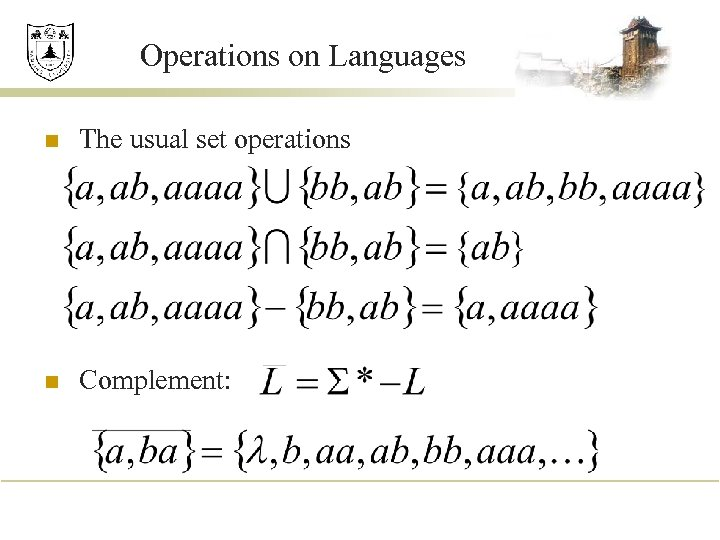Operations on Languages n The usual set operations n Complement: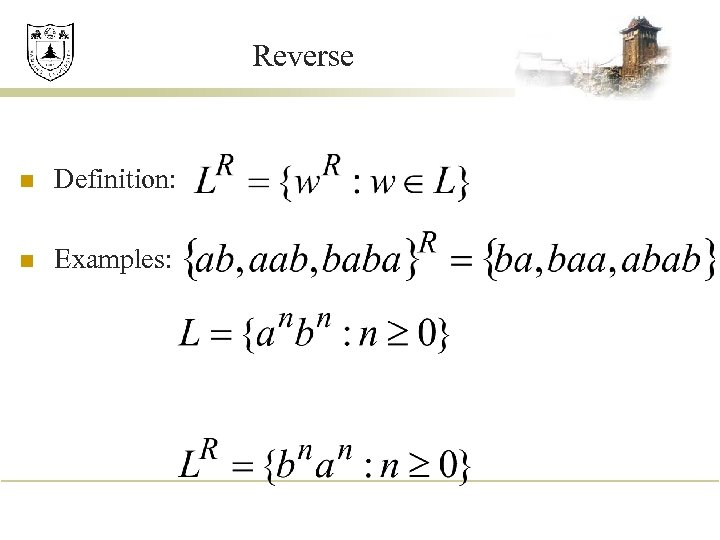Reverse n Definition: n Examples: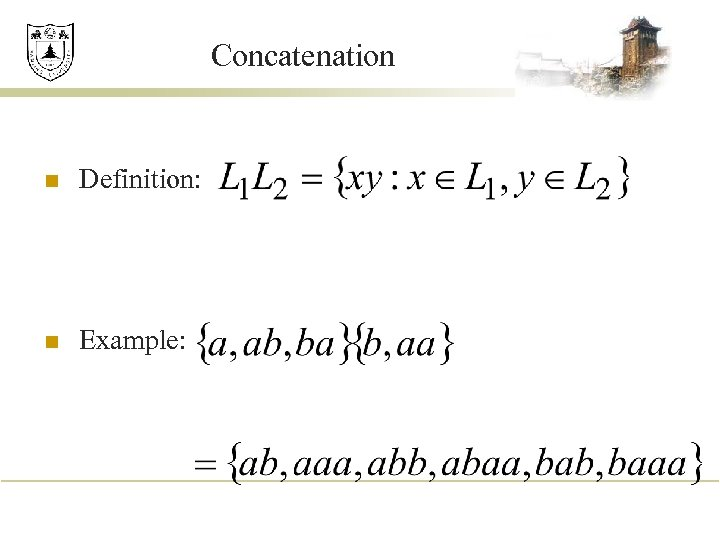Concatenation n Definition: n Example: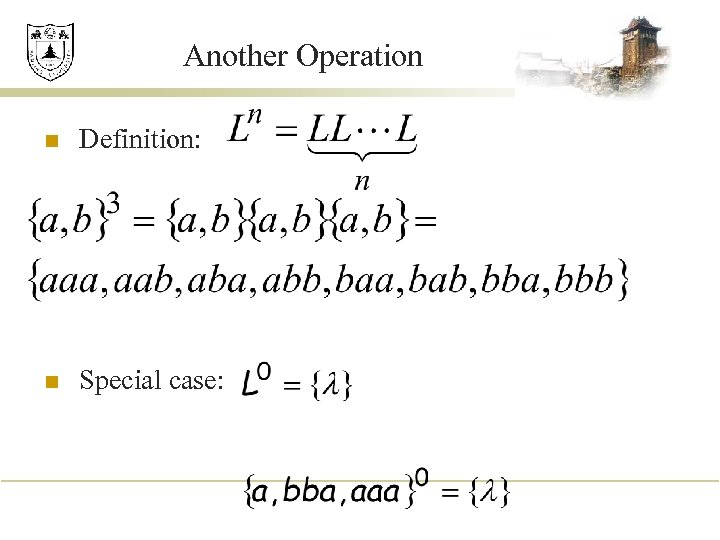Another Operation n Definition: n Special case: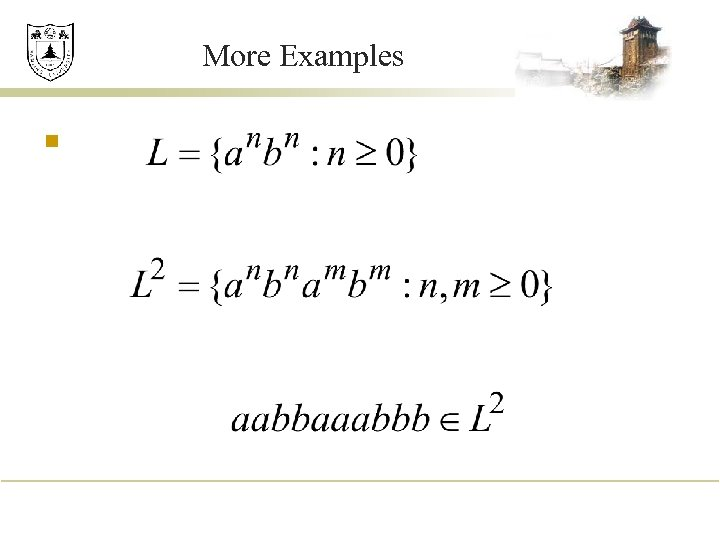More Examples n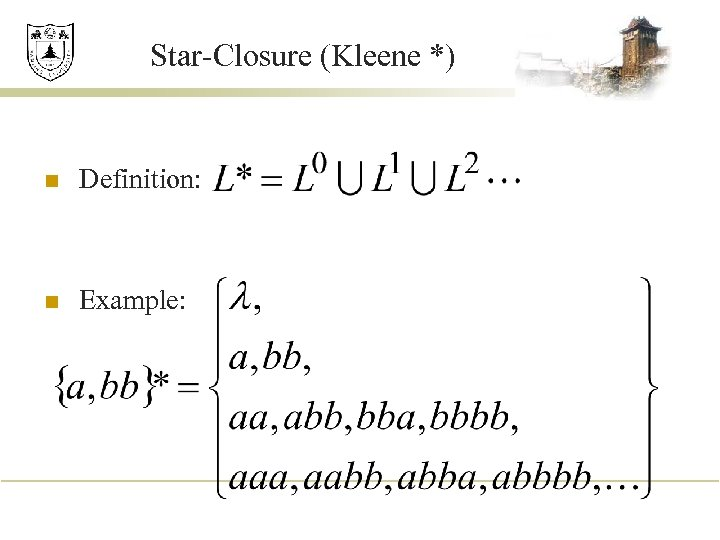Star-Closure (Kleene *) n Definition: n Example: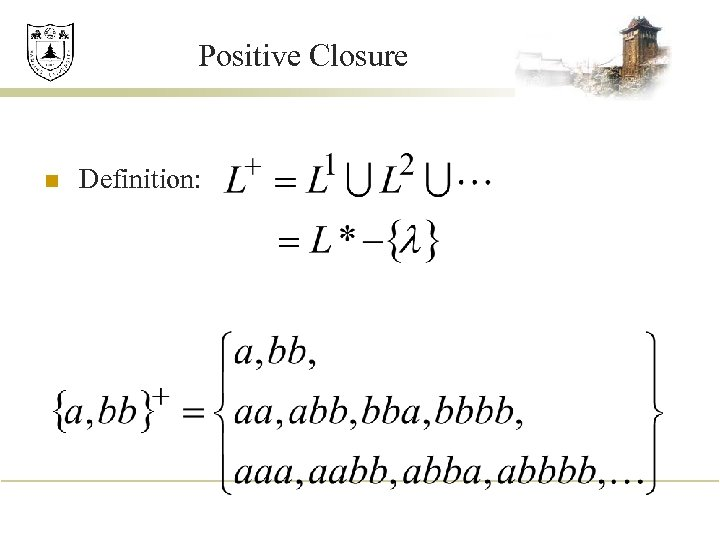Positive Closure n Definition: